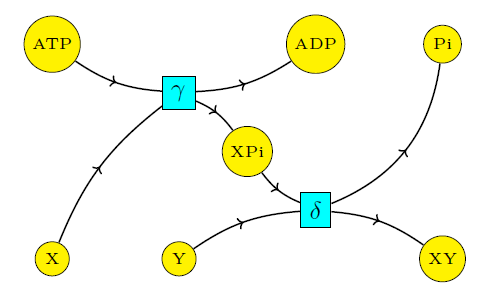## Coupling Through Emergent Conservation Laws (Part 5)

#### John Baez, Jonathan Lorand, Blake Pollard, and Maru Sarazola

Coupling is the way biology makes reactions that 'want' to happen push forward desirable reactions that don't want to happen. Coupling is achieved through the action of enzymes — but in a subtle way. An enzyme can increase the rate constant of a reaction. However, it cannot change the ratio of forward to reverse rate constants, since that is fixed by the difference of free energies, as we saw in Part 2:

$$\displaystyle{ \frac{\alpha_\to}{\alpha_\leftarrow} = e^{-\Delta {G^\circ}/RT} }$$

and the presence of an enzyme does not change this.

Indeed, if an enzyme could change this ratio, there would be no need for coupling! For example, increasing the ratio $$\alpha_\rightarrow/\alpha_\leftarrow$$ in the reaction

$$\mathrm{X} + \mathrm{Y} \mathrel{\substack{\alpha_{\rightarrow} \\\longleftrightarrow\\ \alpha_{\leftarrow}}} \mathrm{XY}$$

would favor the formation of XY, as desired. But this option is not available.

Instead, to achieve coupling, the cell uses enyzmes to greatly increase both the forward and reverse rate constants for some reactions while leaving those for others unchanged!

Let's see how this works. In our example, the cell is trying to couple ATP hydrolysis to the formation of the molecule XY from two smaller parts X and Y. These reactions don't help do that:

$$\begin{array}{cclc} \mathrm{X} + \mathrm{Y} & \mathrel{\substack{\alpha_{\rightarrow} \\\longleftrightarrow\\ \alpha_{\leftarrow}}} & \mathrm{XY} & \qquad (1) \\ \\ \mathrm{ATP} & \mathrel{\substack{\beta_{\rightarrow} \\\longleftrightarrow\\ \beta_{\leftarrow}}} & \mathrm{ADP} + \mathrm{P}_{\mathrm{i}} & \qquad (2) \end{array}$$

but these do:

$$\begin{array}{cclc} \mathrm{X} + \mathrm{ATP} & \mathrel{\substack{\gamma_{\rightarrow} \\\longleftrightarrow\\ \gamma_{\leftarrow}}} & \mathrm{ADP} + \mathrm{XP}_{\mathrm{i}} & (3) \\ \\ \mathrm{XP}_{\mathrm{i}} +\mathrm{Y} & \mathrel{\substack{\delta_{\rightarrow} \\\longleftrightarrow\\ \delta_{\leftarrow}}} & \mathrm{XY} + \mathrm{P}_{\mathrm{i}} & (4) \end{array}$$

So, the cell uses enzymes to make the rate constants for reactions (3) and (4) much bigger than for (1) and (2). In this situation we can ignore reactions (1) and (2) and still have a good approximate description of the dynamics, at least for sufficiently short time scales.

Thus, we shall study quasiequilibria, namely steady states of the rate equation for reactions (3) and (4) but not (1) and (2). In this approximation, the relevant Petri net becomes this:Now it is impossible for $$\mathrm{ATP}$$ to turn into $$\mathrm{ADP} + \mathrm{P}$$ without $$\mathrm{X} + \mathrm{Y}$$ also turning into $$\mathrm{XY}$$. As we shall see, this is the key to coupling!

In quasiequilibrium states, we shall find a nontrivial relation between the ratios $$[\mathrm{XY}]/[\mathrm{X}][\mathrm{Y}]$$ and $$[\mathrm{ATP}]/[\mathrm{ADP}][\mathrm{P}_{\mathrm{i}}]$$. This lets the cell increase the amount of XY that gets made by increasing the amount of ATP present.

Of course, this is just part of the full story. Over longer time scales, reactions (1) and (2) become important. They would drive the system toward a true equilibrium, and destroy coupling, if there were not an inflow of the reactants $$\mathrm{ATP}$$, $$\mathrm{X}$$ and $$\mathrm{Y}$$ and an outflow of the products $$\mathrm{P}_{\mathrm{i}}$$ and $$\mathrm{XY}$$. To take these inflows and outflows into account, we need the theory of 'open' reaction networks... which is something I'm very interested in!

But this is beyond our scope here. We only consider reactions (3) and (4), which give the following rate equation:

$$\begin{array}{ccl} \dot{[\mathrm{X}]} & = & -\gamma_\to [\mathrm{X}][\mathrm{ATP}] + \gamma_\leftarrow [\mathrm{ADP}][\mathrm{XP}_{\mathrm{i}} ] \\ \\ \dot{[\mathrm{Y}]} & = & -\delta_\to [\mathrm{XP}_{\mathrm{i}} ][\mathrm{Y}] +\delta_\leftarrow [\mathrm{XY}][\mathrm{P}_{\mathrm{i}}] \\ \\ \dot{[\mathrm{XY}]} & = &\delta_\to [\mathrm{XP}_{\mathrm{i}} ][\mathrm{Y}] -\delta_\leftarrow [\mathrm{XY}][\mathrm{P}_{\mathrm{i}}] \\ \\ \dot{[\mathrm{ATP}]} & = & -\gamma_\to [\mathrm{X}][\mathrm{ATP}] + \gamma_\leftarrow [\mathrm{ADP}][\mathrm{XP}_{\mathrm{i}} ] \\ \\ \dot{[\mathrm{ADP}]} & =& \gamma_\to [\mathrm{X}][\mathrm{ATP}] - \gamma_\leftarrow [\mathrm{ADP}][\mathrm{XP}_{\mathrm{i}} ] \\ \\ \dot{[\mathrm{P}_{\mathrm{i}}]} & = & \delta_\to [\mathrm{XP}_{\mathrm{i}} ][\mathrm{Y}] -\delta_\leftarrow [\mathrm{XY}][\mathrm{P}_{\mathrm{i}}] \\ \\ \dot{[\mathrm{XP}_{\mathrm{i}} ]} & = & \gamma_\to [\mathrm{X}][\mathrm{ATP}] - \gamma_\leftarrow [\mathrm{ADP}][\mathrm{XP}_{\mathrm{i}} ] -\delta_\to [\mathrm{XP}_{\mathrm{i}}][\mathrm{Y}] +\delta_\leftarrow [\mathrm{XY}][\mathrm{P}_{\mathrm{i}}] \end{array}$$

Quasiequilibria occur when all these time derivatives vanish. This happens when

$$\begin{array}{ccl} \gamma_\to [\mathrm{X}][\mathrm{ATP}] & = & \gamma_\leftarrow [\mathrm{ADP}][\mathrm{XP}_{\mathrm{i}} ]\\ \\ \delta_\to [\mathrm{XP}_{\mathrm{i}} ][\mathrm{Y}] & = & \delta_\leftarrow [\mathrm{XY}][\mathrm{P}_{\mathrm{i}}] \end{array}$$

This pair of equations is equivalent to

$$\displaystyle{ \frac{\gamma_\to}{\gamma_\leftarrow}\frac{[\mathrm{X}][\mathrm{ATP}]}{[\mathrm{ADP}]}=[\mathrm{XP}_{\mathrm{i}} ] =\frac{\delta_\leftarrow}{\delta_\to}\frac{[\mathrm{XY}][\mathrm{P}_{\mathrm{i}}]}{[\mathrm{Y}]} }$$

and it implies

$$\displaystyle{ \frac{[\mathrm{XY}]}{[\mathrm{X}][\mathrm{Y}]} = \frac{\gamma_\to}{\gamma_\leftarrow}\frac{\delta_\to}{\delta_\leftarrow} \frac{[\mathrm{ATP}]}{[\mathrm{ADP}][\mathrm{P}_{\mathrm{i}}]} }$$

If we forget about the species $$\mathrm{XP}_i$$ (whose presence is crucial for the coupling to happen, but whose concentration we do not care about), the quasiequilibrium does not impose any conditions other than the above relation. We conclude that, under these circumstances and assuming we can increase the ratio

$$\displaystyle{ \frac{[\mathrm{ATP}]}{[\mathrm{ADP}][\mathrm{P}_{\mathrm{i}}]} }$$

it is possible to increase the ratio

$$\displaystyle{\frac{[\mathrm{XY}]}{[\mathrm{X}][\mathrm{Y}]} }$$

This constitutes 'coupling'.

We can say a bit more, since we can express the ratios of forward and reverse rate constants in terms of exponentials of free energy differences using the laws of thermodynamics, as explained in Part 2. Reactions (1) and (2), taken together, convert $$\mathrm{X} + \mathrm{Y} + \mathrm{ATP}$$ to $$\mathrm{XY} + \mathrm{ADP} + \mathrm{P}_{\mathrm{i}}$$. So do reactions (3) and (4) taken together. Thus, these two pairs of reactions involve the same total change in free energy, so

$$\displaystyle{ \frac{\alpha_\to}{\alpha_\leftarrow}\frac{\beta_\to}{\beta_\leftarrow} = \frac{\gamma_\to}{\gamma_\leftarrow}\frac{\delta_\to}{\delta_\leftarrow} }$$

But we're also assuming ATP hydrolysis is so strongly exergonic that

$$\displaystyle{ \frac{\beta_\to}{\beta_\leftarrow} \gg \frac{\alpha_\leftarrow}{\alpha_\to} }$$

This implies that

$$\displaystyle{ \frac{\gamma_\to}{\gamma_\leftarrow}\frac{\delta_\to}{\delta_\leftarrow} \gg 1 }$$

Thus,

$$\displaystyle{ \frac{[\mathrm{XY}]}{[\mathrm{X}][\mathrm{Y}]} \gg \frac{[\mathrm{ATP}]}{[\mathrm{ADP}][\mathrm{P}_{\mathrm{i}}]} }$$

This is why coupling to ATP hydrolysis is so good at driving the synthesis of $$\mathrm{XY}$$ from $$\mathrm{X}$$ and $$\mathrm{Y}$$! Ultimately, this inequality arises from the fact that the decrease in free energy for the reaction

$$\mathrm{ATP} \longrightarrow \mathrm{ADP} + \mathrm{P}_{\mathrm{i}}$$

greatly exceeds the increase in free energy for the reaction

$$\mathrm{X} + \mathrm{Y} \longrightarrow \mathrm{XY}$$

But this fact can only be put to use in the right conditions. We need to be in a 'quasiequilibrium' state, where fast reactions have reached a steady state but not slow ones. And we need fast reactions to have this property: they can only turn $$\mathrm{ATP}$$ into $$\mathrm{ADP} + \mathrm{P}_{\mathrm{i}}$$ if they also turn $$\mathrm{X} + \mathrm{Y}$$ into $$\mathrm{XY}$$. Under these conditions, we have 'coupling'.

Next time we'll see how coupling relies on an 'emergent conservation law'.